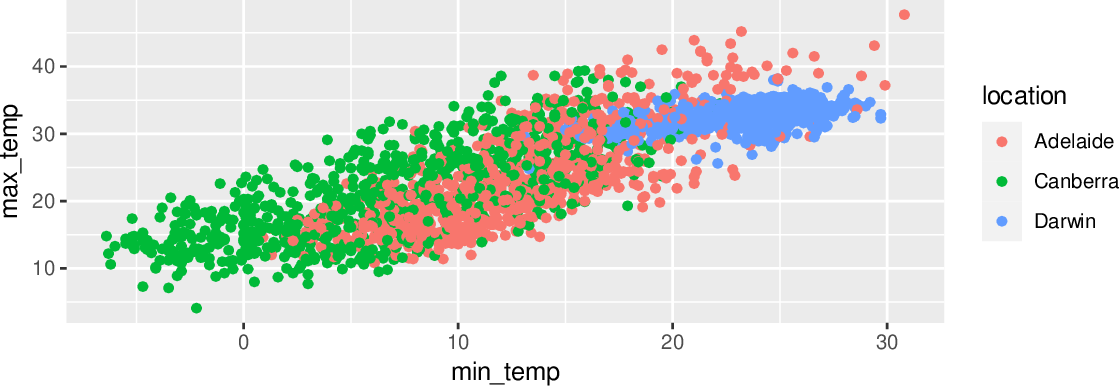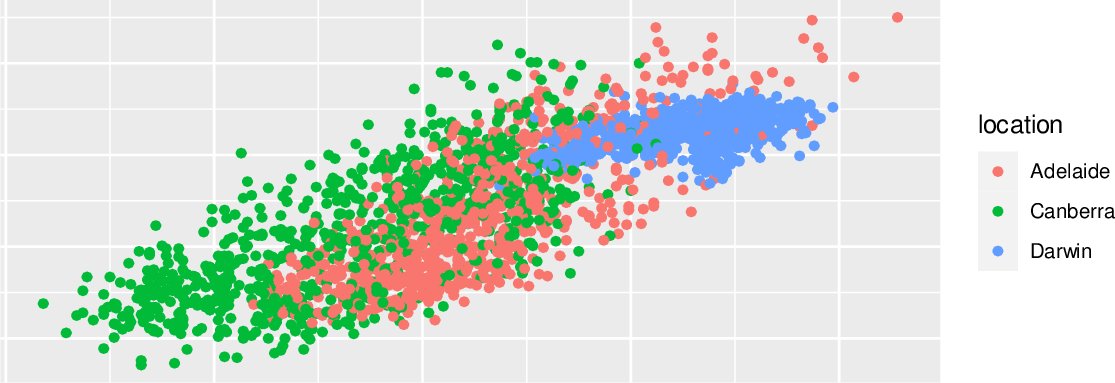Data Science Desktop Survival Guide by Graham WilliamsDesktop Survival Project Home Preface Data Science Introducing R R Constructs R Tasks R Strings R Read, Write, and Create Data Template Data Exploration Data Wrangling Data Visualisation Statistics ML Template ML Scenarios ML Activities ML Applications ML Algorithms Cluster Analysis Decision Trees Computer Vision Graph Data Privacy Literate Data Science Coding with Style Resources Bibliography Index

## Labels Removed

20200317ds %>%   subset(location %in% c("Canberra", "Adelaide", "Darwin")) %>%   sample_frac(0.2) %>%   ggplot(aes(min_temp, max_temp, colour=location)) +   geom_point() +   theme(axis.ticks.x = element_blank(),         axis.text.x  = element_blank(),         axis.title.x = element_blank(),         axis.ticks.y = element_blank(),         axis.text.y  = element_blank(),         axis.title.y = element_blank()) The top plot has default x-axis and y-axis ticks, text, and title. To remove axis labels altogether the bottom plot adds a layer to set the axis ticks, text, and title to ggplot2::element_blank() which is used to draw nothing and to take no space within a non-data component of the plot, using the ggplot2::theme() system: Notice the use of dplyr::sample_frac() to plot a 20% random sample of the dataset and thus the actual points plotted in the two plots above are randomly different.